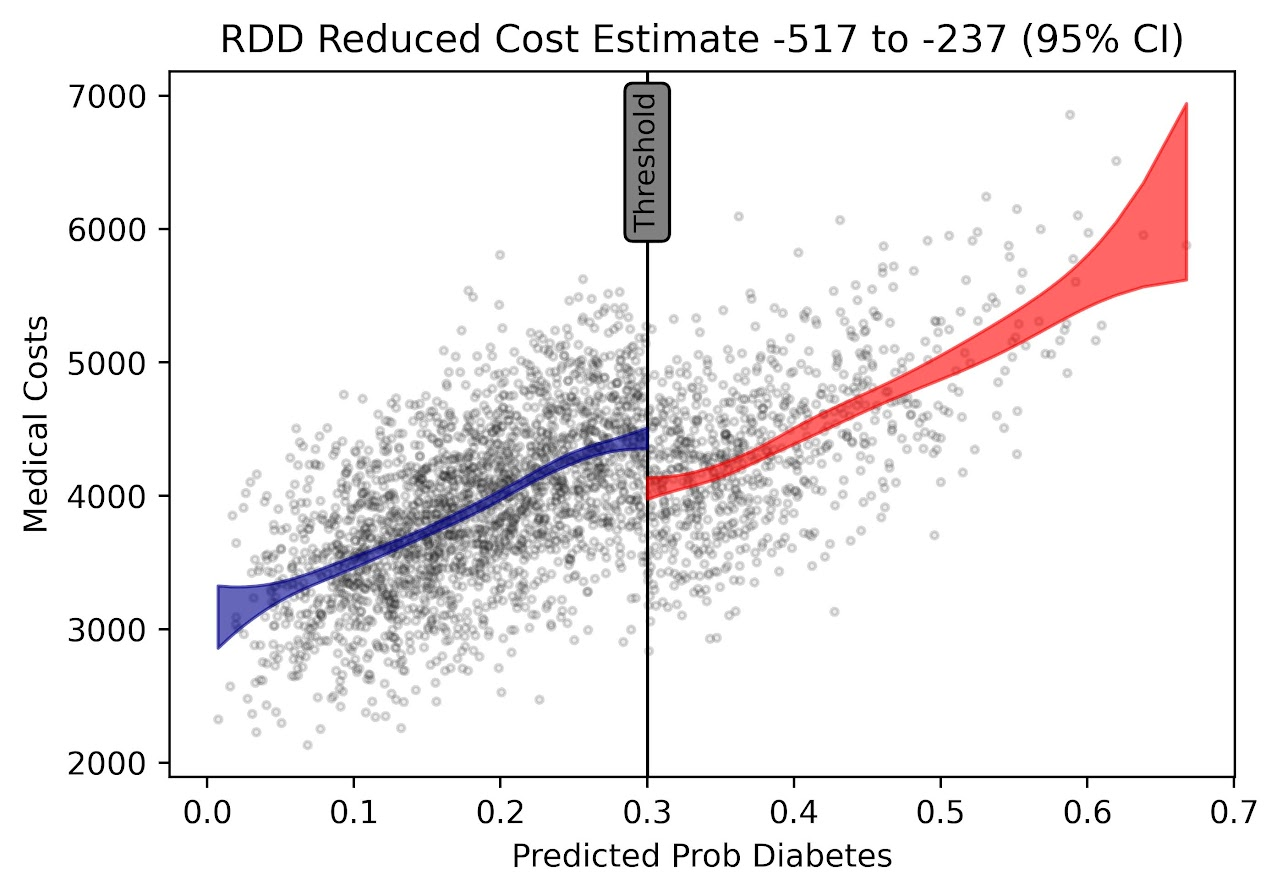# Regression Discontinuity Designs

Regression Discontinuity Designs (RDD) are one way to evaluate predictive model systems with causal outcomes associated with what you do with that information. For a hypothetical example, imagine you have a predictive model assessing the probability that you will be diagnosed with diabetes in the next two years. Those that score above 30% probability get assigned a caseworker, to try to prevent that individual from contracting diabetes. How do you know how effective that caseworker is in reducing diabetes in those high risk individuals?

The RDD design works like this – you have your running variable (here the predicted probability), and the threshold (over 30%) that gets assigned a treatment. You estimate the probability of the actual outcome (it may be other outcomes besides just future diabetes, such as the caseworker may simply reduce overall medical costs even if the person still ends up being diagnosed with diabetes). You then estimate the dip in the predicted outcome just before and just after the threshold. The difference in those two curves is the estimated effect.

Here is an example graph illustrating (with fake data I made, see the end of the post). The bump in the line (going from the blue to the red) is then the average treatment effect of being assigned a caseworker, taking into account the underlying trend that higher risk people here are more likely to have higher medical bills.A few things to note about RDD – so there is a tension between estimating the underlying curve and the counterfactual bump at the threshold. Theoretically values closer to the threshold should be more relevant, so some (see the Wikipedia article linked earlier) try to estimate non-linear weighted curves, giving cases closer to the threshold higher weights. This often produces strange artifacts (that Andrew Gelman likes to point out on his blog) that can miss-estimate the RDD effect. This is clearly the case in noisy data if the line dips just before the threshold and curves up right after the threshold.

So you can see in my code at the end I prefer to estimate this using splines, as opposed to weighted estimators that have a bit of noise. (Maybe someday I will code up a solution to do out of sample predictive evaluations for this as an additional check.) And with this approach it is easy to incorporate other covariates (and look at treatment heterogeneity if you want). Note that while wikipedia says the weighted estimator is non-parametric this is laughably wrong (it is two straight lines in their formulation, a quite restrictive for actually) – while I can see some theoretical justification for the weighted estimators, in practice these are quite noisy and not very robust to minor changes in the weights (and you always need to make some parametric assumptions in this design, both for the underlying curve as well as the standard error around the threshold bump).

There are additional design details you need to be worried about, such as fuzzy treatments or self selection. E.g. if a person can fudge the numbers a bit and choose which side of the line they are on, it can cause issues with this design. In those cases there may be a reasonable workaround (e.g. an instrumental variable design), but the jist of the research design will be the same.

Last but not least, to actually conduct this analysis you need to cache the original continuous prediction. In trying to find real data for this blog post, many criminal justice examples of risk assessment people only end up saving the final categories (low, medium, high) and not the original continuous risk instrument.

If folks have a good public data example that I could show with real data please let me know! Scouting for a bit (either parole/probabation risk assessment, or spatial predictive policing) has not turned up any very good examples for me to construct examples with (also health examples would be fine as well).

This is the simulated data (in python), the RDD graph, and the statsmodel code for how I estimate the RDD bump effect. You could of course do more fancy things (such as penalize the derivatives for the splines), but this I think would be a good way to estimate the RDD effect in many designs where appropriate.

``````from matplotlib import pyplot as plt
import numpy as np
import pandas as pd
import statsmodels.api as sm
import statsmodels.formula.api as smf

########################################
# Simulating data
np.random.seed(10)
n_cases = 3000 # total number of cases
# pretend this is predicted prob
prob = np.random.beta(3,10,size=n_cases)
# pretend this is med costs over a year
med_cost = 3000 + 5000*prob + -500*(prob > 0.3) + np.random.normal(0,500,n_cases)
df = pd.DataFrame(zip(prob,med_cost), columns=['Prob','MedCost'])
# could do something fancier with non-linear effects for prob
########################################

########################################
# Fitting regression model

# Knots are small distance from threshold
# (Could also do a knot right on threshold)
mod = smf.ols(formula='MedCost ~ bs(Prob,knots=[0.2,0.25,0.35,0.4]) + I(Prob > 0.3)', data=df)
res = mod.fit()
print(res.summary())
########################################

########################################
# Plotting fit

# Getting standard errors
prob_se = res.get_prediction().summary_frame()
prob_se['Prob'] = prob
prob_se.sort_values(by='Prob',inplace=True,ignore_index=True)
low = prob_se[prob_se['Prob'] <= 0.3].copy()
high = prob_se[prob_se['Prob'] > 0.3].copy()

# Getting effect for threshold bump
coef = res.summary2().tables
ci = coef.iloc[1,4:6].astype(int).to_list()

fig, ax = plt.subplots(figsize=(6,4))
ax.scatter(df['Prob'], df['MedCost'], c='grey',
edgecolor='k', alpha=0.15, s=5, zorder=1)
ax.axvline(0.3, linestyle='solid', alpha=1.0,
color='k',linewidth=1, zorder=2)
ax.fill_between(low['Prob'],low['mean_ci_lower'],
low['mean_ci_upper'],alpha=0.6,
zorder=3, color='darkblue')
ax.fill_between(high['Prob'],high['mean_ci_lower'],
high['mean_ci_upper'],alpha=0.6,
zorder=3, color='red')
ax.set_xlabel('Predicted Prob Diabetes')
ax.set_ylabel('Medical Costs')
ax.set_title(f'RDD Reduced Cost Estimate {ci} to {ci} (95% CI)')
ax.text(0.3,6500,'Threshold',rotation=90, size=9,
ha="center", va="center",
bbox=dict(boxstyle="round", ec='k',fc='grey'))
plt.savefig('RDD.png', dpi=500, bbox_inches='tight')
########################################``````